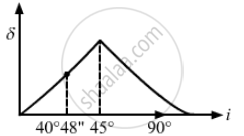Advertisement Remove all ads

# Light is Incident from Glass (μ = 1.5) to Air. Sketch the Variation of the Angle of Deviation δ with the Angle of Incident I for 0 < I < 90°. - Physics

Short Note

Light is incident from glass (μ = 1.5) to air. Sketch the variation of the angle of deviation δ with the angle of incident i for 0 < i < 90°.

Advertisement Remove all ads

#### Solution

Given,
Refractive index of glass, μg = 1.5
Refractive index of air, μa= 1.0
Angle of incidence 0° < i < 90
Let us take θc as the Critical angle
$\Rightarrow \frac{\sin \theta c}{\sin r} = \frac{\mu_a}{\mu_g}$

$\Rightarrow \frac{\sin \theta_c}{\sin 90^\circ } = \frac{1}{1 . 5} = 0 . 66$

$\Rightarrow \sin \theta_c = 0 . 66$

$\Rightarrow \theta_c = \sin {}^{- 1} \left( 0 . 66 \right)$
⇒ θc = 40°48"The angle of deviation (δ) due to refraction from glass to air increases as the angle of incidence increases from 0° to 40°48". The angle of deviation (δ) due to total internal reflection further increases from 40°48" to 45° and then it decreases, as shown in the graph.

Is there an error in this question or solution?
Advertisement Remove all ads

#### APPEARS IN

HC Verma Class 11, 12 Concepts of Physics 1
Chapter 18 Geometrical Optics
Q 29 | Page 414
Advertisement Remove all ads

#### Video TutorialsVIEW ALL 

Advertisement Remove all ads
Share
Notifications

View all notifications

Forgot password?